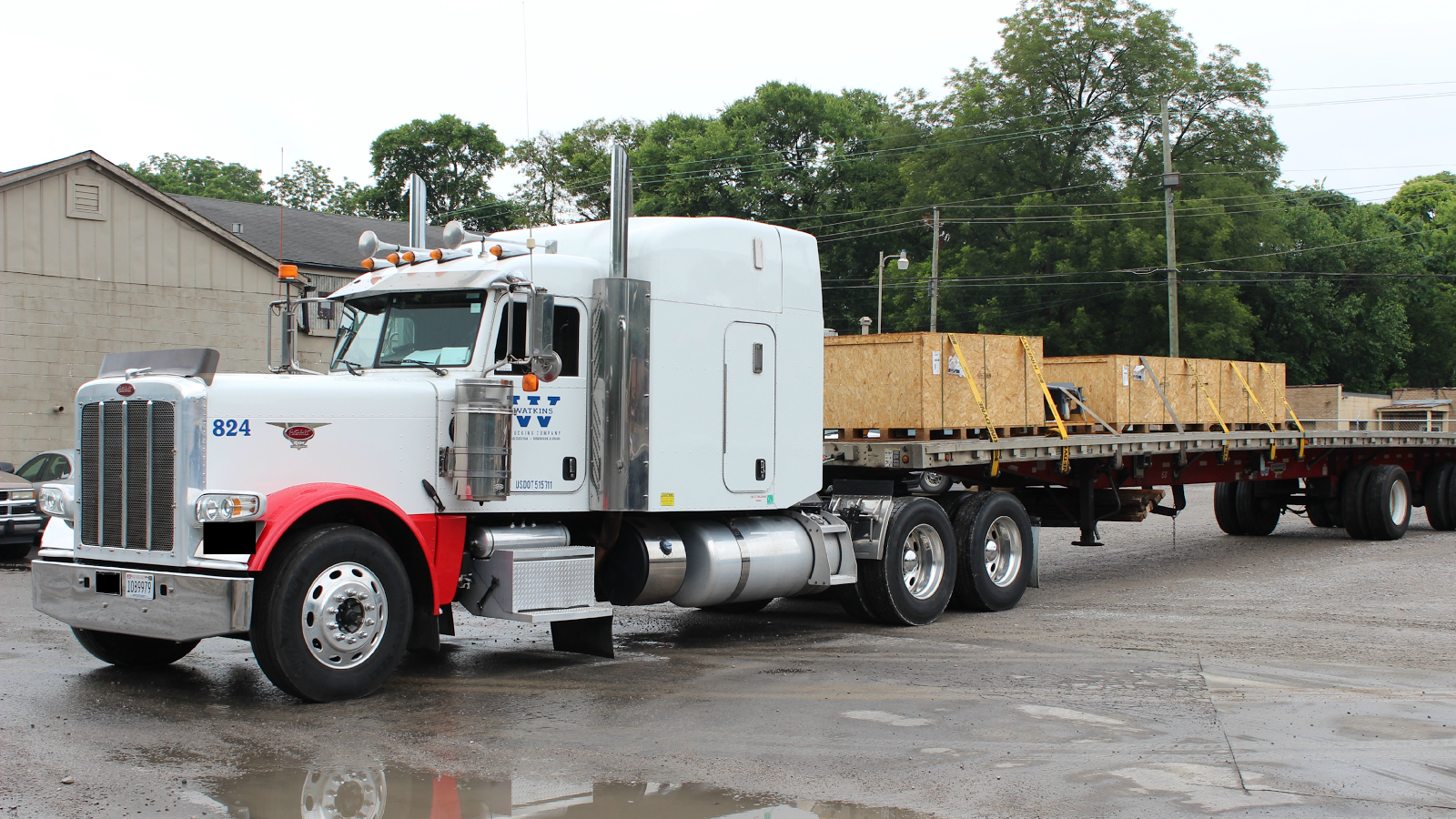## 20171003

### Physics quiz question: comparing directions and magnitudes of forces

Physics 205A Quiz 3, fall semester 2017
Cuesta College, San Luis Obispo, CA"PACCAR Financial - Watkins Trucking 1"
TruckPR
flic.kr/p/oMJDhgA 600 kg load of cargo rests on the bed of a 8,850 kg stationary truck[*]. Newton's __________ law tells you that these two forces are equal in magnitude and opposite in direction:
Normal force of ground on the truck.
Normal force of truck on the cargo load.
(A) first.
(B) second.
(C) third.
(D) (These forces are not equal in magnitude and/or opposite in direction.)

[*] trucktrend.com/truck-reviews/163-0501-gm-kodiak-topkick-4x4/.

Correct answer (highlight to unhide): (D)

The cargo load has two forces acting on it:
Weight force of Earth on the cargo load (mcargo·g = 5.9×103 N, downwards).
Normal force of truck on the cargo load (upwards).
Because the cargo load is stationary, these two forces are equal in magnitude (each 5.9×103 N) and opposite in direction, due to Newton's first law, so the upwards normal force of truck on the cargo load must be 5.9×103 N in magnitude.

The truck has three forces acting on it:
Weight force of Earth on the truck (mtruck·g = 8.67×104 N, downwards).
Normal force of cargo load on the truck (downwards).
Normal force of ground on the truck (upwards).
Because the direction of the normal force of ground on the truck is already known to be upwards, and the direction of the normal force of truck on the cargo load is also known to be upwards, then they cannot be forces that are opposite in direction.

(To determine magnitudes of the two forces in question, because the truck is stationary, the magnitudes of the two downward forces added together must equal the magnitude of the upwards force, due to Newton's first law. From Newton's third law, the downwards normal force of the cargo load on the truck must be equal in magnitude and opposite in direction to the upwards normal force of the truck on the cargo load, so they are each 5.9×103 N.)

(Subsequently, the upwards normal force of the ground on the truck will be equal to the addition of the downwards force of the weight force of Earth on the truck (8.67×104 N) with the downwards normal force of the cargo load on the truck (5.9×103 N), and thus is 9.26×104 N.)

(Then since the magnitude of the normal force of ground on the truck is 9.26×104 N, and the magnitude of the normal force of truck on the cargo load is 5.9×103 N, then they cannot be forces that have the same magnitude, as well.)

Sections 70854, 70855
Exam code: quiz03T4uC
(A) : 8 students
(B) : 2 students
(C) : 10 students
(D) : 34 students

Success level: 63%
Discrimination index (Aubrecht & Aubrecht, 1983): 0.81